# Inverse Operations: Addition and Subtraction#### The Inverse of Addition is Subtraction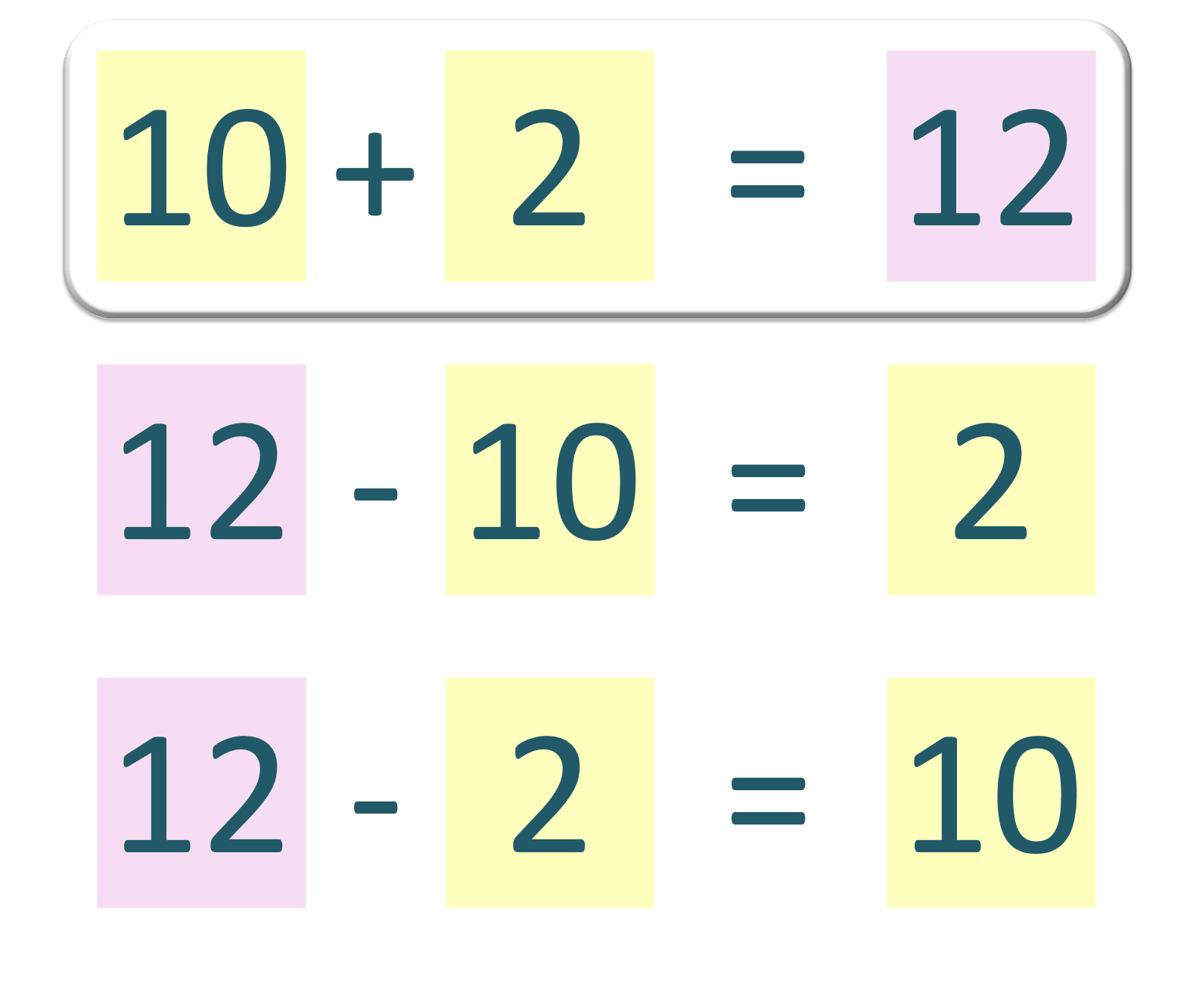• In maths, inverse means opposite.
• Subtraction is the inverse of addition, which means that subtraction “undoes” addition.
• We can rearrange the numbers in an addition sentence to make two different subtraction sentences.
• 10 + 5 = 15 can be written as 15 – 10 = 5 or 15 – 5 = 10.
• The answer to an addition can be written at the start of a subtraction sentence.
• The two numbers that are added together in an addition go after the subtraction sign and after the equals sign in either order.
• If you know an addition, then you can simply use this rule to rearrange the numbers to form a subtraction.

#### The Inverse of Subtraction is Addition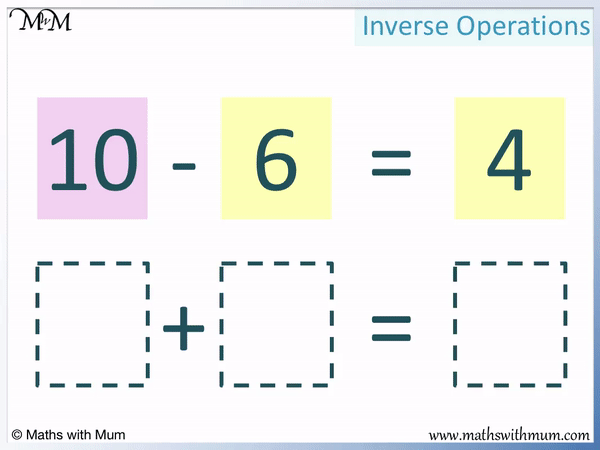• We can rearrange the numbers in a subtraction sentence to make two different addition sentences.
• 10 – 6 = 4 can be written as 6 + 4 = 10 or 4 + 6 = 10.
• The number at the start of a subtraction that we are subtracting from becomes the answer of an addition sentence.
• The two other numbers in the subtraction are the two numbers that get added together in an addition sentence.
• If you know a subtraction, then you can simply use this rule to rearrange the numbers to form an addition.

Addition and subtraction are inverse operations.

The numbers can be rearranged in an addition to form a subtraction and vice versa.• We will use inverse operations to write the addition of 25 + 8 = 33 as a subtration.
• If you know an addition fact, there is no need to do any calculations, simply rearrange the numbers to write it as a subtraction.
• To write an addition as a subtraction, write the answer of the addition at the start of the subtraction.
• 33 is the answer to the addition and so, we write the 33 at the start of the subtraction.
• The two numbers being added together in an addition are then placed after the subtraction sign and the equals sign in any order.
• The two numbers being added together are 25 and 8.
• We can write the 25 after the subtraction sign and the 8 after the equals sign.
• 25 + 8 = 33 can be written as 33 – 25 = 8.
• We can also write 25 + 8 = 33 as 33 – 8 = 25.Supporting Lessons

#### Writing Subtractions as Additions; Further Examples# Inverse Operations of Addition and Subtraction

## What is the Inverse of Addition?

The inverse of addition is subtraction. This means that subtraction is the opposite of addition. Adding and subtracting the same number cancel each other out. For example, adding 2 and then subtracting 2 results in no change to the original number.

Subtraction is the inverse of addition.An inverse operation is a calculation which has the effect of ‘undoing’ another calculation.

Here is the addition of 10 + 2 = 12.We started with the number 10 and added 2 to it to get 12.

We can subtract 2 from 12 to get back to 10.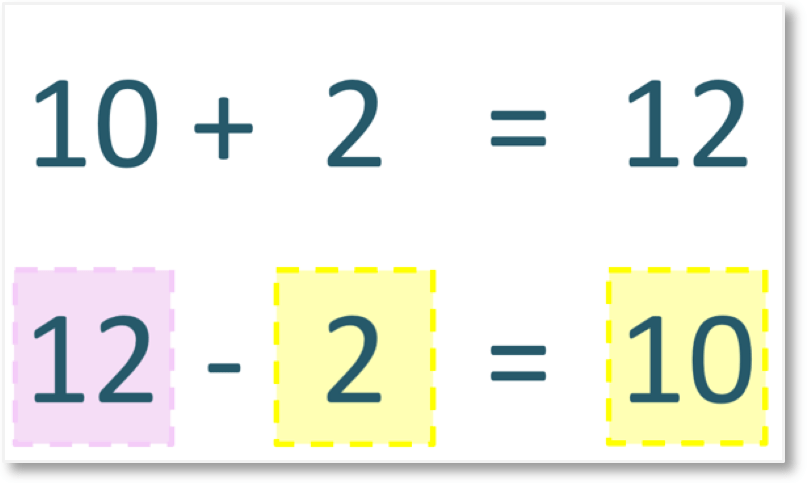Adding and subtracting 2 have the effect of cancelling each other out. We started with 10 and after adding 2 and then subtracting 2, we ended up back at 10.

Adding and subtracting the same number have the effect of cancelling each other out. They are inverse operations. This is true only if the number being subtracted is the same as the number being added.

## How to Write Addition as Subtraction

1. Write the answer of the addition as the number at the start of the subtraction, before the subtraction sign.
2. Write the two numbers being added in the addition after the subtraction sign and the equals sign of the subtraction sentence in either order.

For example, here is 10 + 5 = 15. We will write this addition sentence as a subtraction sentence.Step 1 is to write the answer of the addition as the number at the start of the subtraction, before the subtraction sign.

The answer of the addition in 10 + 5 = 15 is the number 15. We write this number first, before the subtraction sign.

Step 2 is to write the two numbers being added in the addition after the subtraction sign and the equals sign of the subtraction sentence in either order.

The two numbers being added in the addition are 10 and 5.

We write these numbers after the subtraction sign and the equals sign in either order.

For every addition sentence, we can write two different subtraction sentences.

10 + 5 = 15 can be written as 15 – 10 = 5 or 15 – 5 = 10. The numbers of 5 and 10 can be written in either order to make two different subtractions.

A subtraction sentence can be written by simply rearranging the numbers in an addition sentence. If the numbers in the addition sentence are known, there is no need to do any calculations to turn it into a subtraction. Simply rearrange the numbers.

For example, here is the addition of 39 + 26 = 65.

The subtraction sentence can be written without any need to do a calculation.

The first step is to write the answer to the addition as the first number of the subtraction, before the subtraction sign.

We write 65 at the start of the subtraction.The second step is to write the two numbers being added after the subtraction sign and after the equals sign respectively.

We write 39 after the subtraction sign and 26 after the equals sign.

39 + 26 = 65 can be rewritten as a subtraction as 65 – 39 = 26.

The numbers of 39 and 26 can be switched to create the second subtraction sentence.39 + 26 = 65 can be rewritten as a subtraction as 65 – 26 = 39.

We did perform any calculations to write these subtractions. We simply rearranged the numbers in the known addition sentence that we were given.When teaching the writing of subtraction sentences from addition sentences, it is important to show the two different subtractions that can be made, comparing the positions of the numbers.

## What is the Inverse of Subtraction?

The inverse of subtraction is addition. This means that subtraction is the opposite of addition. Subtracting and adding the same number have the effect of cancelling each other out. For example, subtracting 5 and adding 5 to a number does not change the size of that number.Here is 10 – 2 = 8.

We started with 10 and subtracted 2 to get to 8.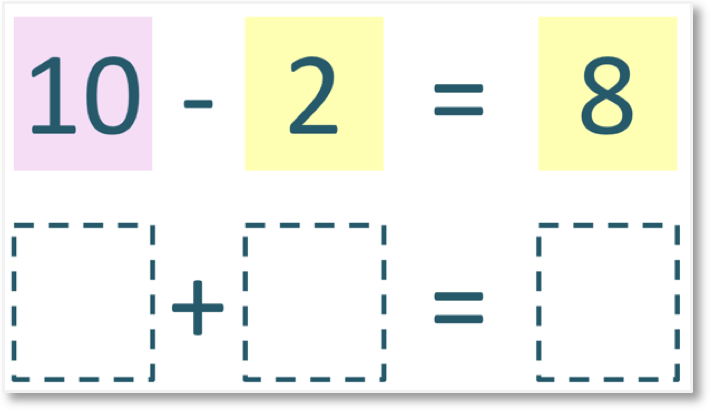The opposite of subtracting 2 is to add 2.

We can add 2 to 8 to get back to 10.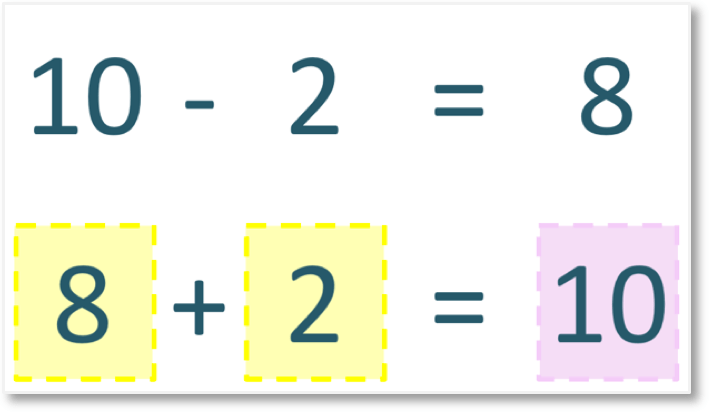Subtracting 2 and adding 2 are inverse operations. We started at 10 and when we subtracted 2 and then added 2, we arrived back at 10.

Subtracting and adding the same number are inverse operations.

## How to Write Subtraction as Addition

1. Write the number before the subtraction sign as the answer at the end of the addition, after the equals sign.
2. Write the other two numbers in the subtraction as the two numbers added together, either side of the addition sign.

For example, here is the subtraction of 20 – 5 = 15. We will use the steps shown to write it as an addition.

The first step is to write the number before the subtraction sign as the answer at the end of the addition, after the equals sign.

The number before the subtraction sign is 20. We will write this as the answer of our addition.The second step is to write the other two numbers in the subtraction as the two numbers added together, either side of the addition sign.

The other two numbers are 5 and 15. We write this on either side of the addition sign.

The subtraction of 20 – 5 = 15 can be rewritten as an addition as 5 + 15 = 20.

The numbers of 5 and 15 can be written in the other order to create another addition sentence.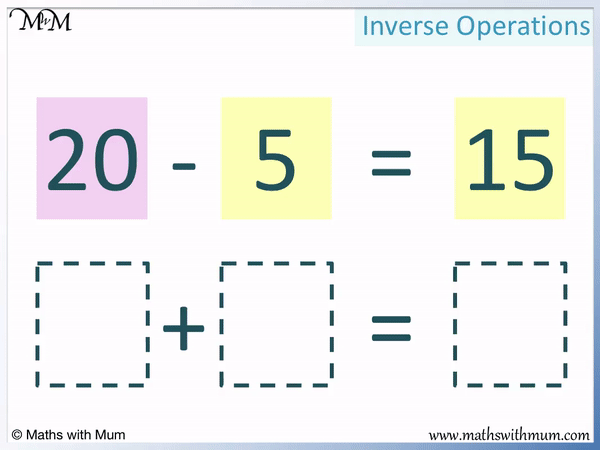The subtraction of 20 – 5 = 15 can be rewritten as an addition as 15 + 5 = 20.It does not matter how large the numbers are in the addition or subtraction because there is no need to perform any calculations if the original subtraction sentence is known. We simply need to rearrange the numbers

For example, here is 53 – 17 = 36. We will rearrange the numbers to form two different addition sentences.

The first step is to write the number at the start of the subtraction at the end of the addition sentence after the equals sign.The subtraction of 53 – 17 = 36 is written as an addition as 17 + 36 = 53.

We can switch the places of these these two numbers to create another addition sentence.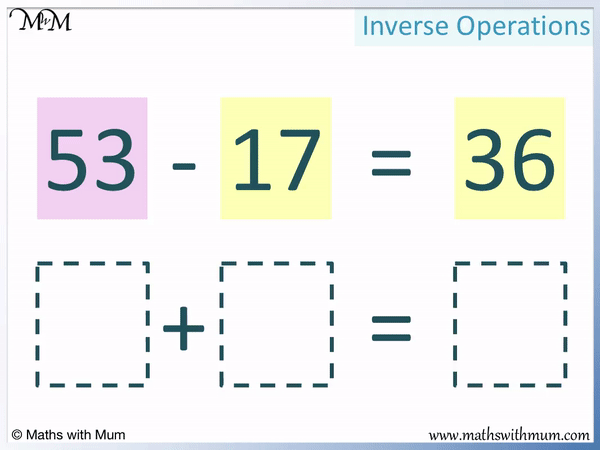The subtraction of 53 – 17 = 36 is written as an addition as 36 + 17 = 53.Now try our lesson on Inverse Operations: Multiplication and Division where we learn the relationship between multiplication and division.error: Content is protected !!